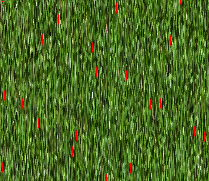# Xojo Graphic Overlay - What am I doing Wrong?

I have a large picture with areas masked out in Magenta (&cff00ff)
I have another image (smaller) that is of “grass”
I am trying to replace the magenta areas of the 1st picture with the grass from the second, leaving the NON-magenta parts of the 1st pic as-is

``````Dim grass As picture
grass=picture.Open(GetFolderItem("").child("grass.png"))
Dim grs As RGBSurface = grass.RGBSurface
Dim prs As RGBSurface = newp.RGBSurface
Dim x As Integer
Dim y As Integer
Dim x1 As Integer
Dim y1 As Integer
For x=0 To newp.Width
For y=0 To newp.Height
If prs.Pixel(x,y)=&cff00ff Then prs.pixel(x,y)=grs.pixel(x1,y1)
y1=y1+1
If y1=grass.height Then y1=0

Next y
x1=x1+1
If x1=grass.width Then x1=0
Next x
``````

the aspect is all screwed… as a text I took the “grass” and drew a RED “X”, so that the finished image should show that same X, but here is what I get (this is just a small sample cut from the much larger image)still would like to know if anyone sees my flaw.
in the mean time I just made the grass image bigger than the main image so the coordinates could be 1 to 1, and it worked

I find code MUCH easier to follow with proper naming:

Never used RGBsurface, so:

Maybe use picSurface.Height and .Width?
What’s the bit depth of the picture?
Alpha Channel?

… and maybe -1?

``````For picX = 0 To newp.Width - 1
For picY = 0 To newp.Height -1
``````

no, it has something to do whe grassX and grassY… I just made a larger version of grass and took those out, and it worked perfectly

You forgot to reset y1 before starting each nested FOR.

``````For x=0 To newp.Width
y1=0
For y=0 To newp.Height``````

Your code only works correct if the larger picture is a multiple size of grass? Otherwise the proportions get skewed.